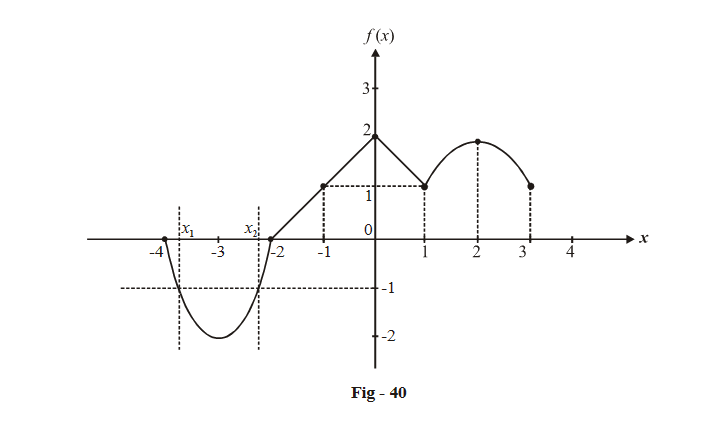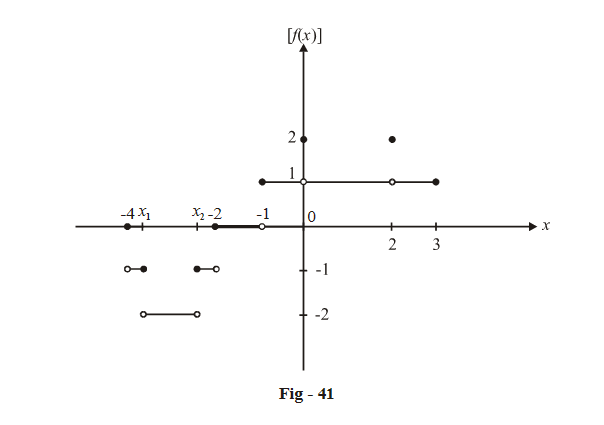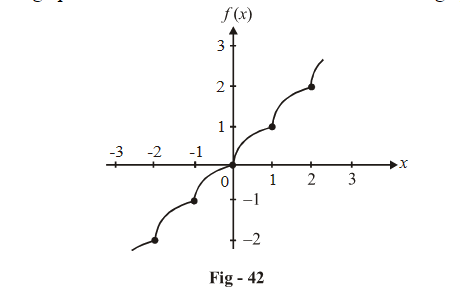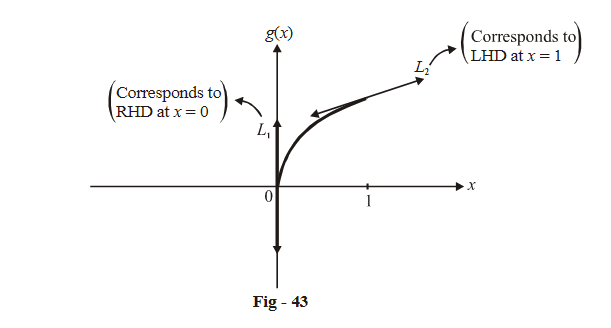# Limits Continuity Differentiability And Differentiation Set-7

Go back to  'SOLVED EXAMPLES'

Example – 17

Let  f\left( x \right) = \left\{ {\begin{align}{}{x + a}\qquad{,x < 0}\\{\left| {x - 1} \right|}\qquad{,x \ge 0}\end{align}} \right\}\,{\rm{and \; }}g\left( x \right) = \left\{ {\begin{array}{*{20}{c}}{x + 1}\qquad\qquad{,x < 0}\\{{{\left( {x - 1} \right)}^2} + b}\qquad{,x \ge 0}\end{array}} \right\}

where a and b are non-negative real numbers. Determine the composite function g(f (x)). If g(f (x)) is continuous for all real x, determine the values of a and b. For these values of a and b, will g(f (x)) be differentiable at   x = 0?

Solution:   Evaluation of the composition of piecewise defined functions can be tricky; hence follow the solution to this problem carefully.

g\left( {f\left( x \right)} \right) = \left\{ {\begin{align} &{f\left( x \right) + 1}&{f\left( x \right) < 0}\\&{{{\left( {f\left( x \right) - 1} \right)}^2} + b}&{f\left( x \right) \ge 0}\end{align}} \right\}

The conditions ‘f (x) < 0’ and ‘ $$f\left( x \right) \ge 0$$ ’ have to be written in terms of x.

Notice from the definition of f (x) that f (x) < 0 only when x + a < 0 i.e.

$$f\left( x \right) < 0 \Rightarrow x < - a.$$

so, $$f\left( x \right) \ge 0\,{\rm{when }}x \ge - a$$  . But also notice that the definition of f (x) changes at x = 0.

Hence, g(f (x)) can be rewritten as.

g\left( {f\left( x \right)} \right) = \left\{ {\begin{align}&{f\left( x \right) + 1}&{x < - a}\\&{{{\left( {f\left( x \right) - 1} \right)}^2} + b}&{x \ge - a}\end{align}} \right\}

= \left\{ {\begin{align}&{x + a + 1}&{x < - a}\\&{{{\left( {x + a - 1} \right)}^2} + b}&{ - a \le x < 0}\\&{{{\left( {\left| {x - 1} \right| - 1} \right)}^2} + b}&{x \ge 0}\end{align}} \right\}

= \left\{ {\begin{align}&{x + a + 1}&{x < - a}\\ &{{{\left( {x + a - 1} \right)}^2} + b}&{ - a \le x < 0}\\&{{x^2} + b}&{0 \le x < 1}\\&{{{\left( {x - 2} \right)}^2} + b}&{1 \le x}\end{align}} \right\}

This is the ‘simplified’ definition of g(f (x)). Reread the whole discussion above carefully till you fully understand it.

Now our task is easy. We just need to equate LHL and RHL at each of the critical points x = –a, 0, 1 to find out a and b.

The remaining part of this question is left as an exercise to you. The answers are:

a = 1                b = 0

For these values, f (x) is non-differentiable at $$x = \pm 1$$

Example - 18

Let f (x) be the function (defined in Example -14, Section-3)

f\left( x \right) = \left\{ {\begin{align}&{2{x^2} + 12x + 16}&,&{ - 4 \le x \le - 2}\\&{2 - \left| x \right|}&,&{ - 2 < x \le 1}\\&{4x - {x^2} - 2}&,&{1 < x \le 3}\end{align}} \right\} . Plot [f (x)] and discuss its continuity.

Solution:   We drew the graph of this function in Fig. 29. We are replicating it in more detail here:To draw the required graph, notice that the value of [f (x)] will change every time (the value of ) f(x) crosses an integer.

For example, notice from the graph the following facts:

When $$x \in \left( { - 4,{x_1}} \right],0 < f\left( x \right) \le - 1 \Rightarrow \left[ {f\left( x \right)} \right] = - 1$$

When $$x \in \left( {{x_1},{x_2}} \right), - 2 \le f\left( x \right) < - 1 \Rightarrow \left[ {f\left( x \right)} \right] = - 2$$

When $$x = 0\,\,\,\,{\rm{or }}x = 2,f\left( x \right) = 2 \Rightarrow \left[ {f\left( x \right)} \right] = 2$$

and so on.

xand x2 can be evaluated by solving

\begin{align}&f\left( x \right) = 2{x^2} + 12x + 16 = - 1\\& \Rightarrow 2{x^2} + 12x + 17 = 0\\ &\Rightarrow \qquad x = - 3 \pm \frac{{\sqrt {10} }}{4}\\ & \Rightarrow {x_1} = - 3 - \frac{{\sqrt {10} }}{4},{x_2} = - 3 + \frac{{\sqrt {10} }}{4}\end{align}

For the sake of completeness, the complete definition of [f (x)] is given below :

\left[ {f\left( x \right)} \right] = \left\{ {\begin{align}&{\;\;0 }\qquad\qquad\qquad{x = - 4}\\&{ - 1}\qquad\qquad{ - 4 < x \le {x_1}}\\&{- 2 }\qquad\qquad{{x_1} < x < {x_2}}\\&{- 1 }\qquad\qquad{{x_2} \le x < - 2}\\&\;\;0\qquad\qquad{ - 2 \le x < - 1}\\&\;\;1\qquad\qquad{ - 1 \le x < 0}\\&\;\;2\qquad\qquad{x = 0}\\&\;\;1\qquad\qquad{0 < x < 2}\\&2\qquad\qquad{\;\;x = 2}\\&1\qquad\qquad{2 < x \le 3}\end{align}} \right\}

The graph of [f (x)] is:This function is discontinuous at the following points:

$$x = - 4,\,\,{x_1},\,\,{x_2},\,\, - 2,\,\, - 1,\,\,0,\,\,2$$

Example – 19

Let  $$\alpha \in \mathbb{R}.$$ Prove that a function $$f:\mathbb{R} \to \mathbb{R}$$  is differentiable at $$\alpha$$  if and only if there exists a function $$g:\mathbb{R} \to \mathbb{R}$$  which is continuous at $$\alpha$$ and satisfies  $$f\left( x \right) - f\left( \alpha \right) = g\left( x \right)\left( {x - \alpha } \right)$$ for all $$x \in \mathbb{R}$$ .

Solution:   The proof that we seek is two-way that is, when we say that

(P is true) if and only if (Q is true)

we mean that

(P is true) implies (Q is true)

and (Q is true)implies (P is true).

For this question, we first assume the existence of a function g (x) where g (x) satisfies

$$f\left( x \right) - f\left( \alpha \right) = g\left( x \right)\left( {x - \alpha } \right)$$  and g(x) is continuous at $$x = \alpha .$$

Due to this continuity,

$$\mathop {\lim }\limits_{x \to \alpha } g\left( x \right){\rm{ exists}}$$

But,

$$\mathop {\lim }\limits_{x \to \alpha } g\left( x \right) = \mathop {\lim }\limits_{x \to \alpha } \frac{{f\left( x \right) - f\left( \alpha \right)}}{{x - \alpha }}$$

\begin{align}& = \mathop {\lim }\limits_{h \to 0} \frac{{f\left( {\alpha + h} \right) - f\left( \alpha \right)}}{h}\\ &= f'\left( \alpha \right)\end{align}

Hence, $$f'\left( \alpha \right)$$  exists or f (x) is differentiable at x = a.

The other way proof is left as an exercise to the reader

Example – 20

Evaluate the differentiability of   $$f\left( x \right) = \left[ x \right] + \sqrt {\left\{ x \right\}}$$

Solution:   We drew the graph of this function in the unit on Functions. That graph is replicated here:f (x) is obviously continuous everywhere but non-differentiable at all integer points. Let us evaluate the LHD and RHD at each integer. For that, we analyse the segment of the curve between any two adjacent integers. Lets pick up the segment between 0 and 1; this segment is part of the segment $$f\left( x \right) = \sqrt x :$$The lines L1 and L2 correspond to the RHD at x = 0 and LHD at x = 1 respectively.

RHD(at x = 0) $$= \mathop {\lim }\limits_{h \to 0} \frac{{g\left( {0 + h} \right) - g\left( 0 \right)}}{h}$$

\begin{align} & =\underset{h\to 0}{\mathop{\lim }}\,\left\{ \frac{\sqrt{h}-0}{h} \right\} \\ &=\underset{h\to 0}{\mathop{\lim }}\,\frac{1}{\sqrt{h}} \\ & =\infty \left( This~tangent~is\text{ }vertical \right). \\ \end{align}

LHD (at x = 1) $$= \mathop {\lim }\limits_{h \to 0} \frac{{g\left( {1 - h} \right) - g\left( 1 \right)}}{h}$$

\begin{align}& = \mathop {\lim }\limits_{h \to 0} \frac{{\sqrt {1 - h} - 1}}{{ - h}}\\ &= \mathop {\lim }\limits_{h \to 0} \frac{{ - h}}{{ - h\left( {\sqrt {1 - h} + 1} \right)}}\left( {By{\rm{ }}\,rationalization} \right)\\& = \frac{1}{2}\end{align}

Therefore LHD at each integer is  and RHD at each integer is $$\infty$$

Learn from the best math teachers and top your exams

• Live one on one classroom and doubt clearing
• Practice worksheets in and after class for conceptual clarity
• Personalized curriculum to keep up with school